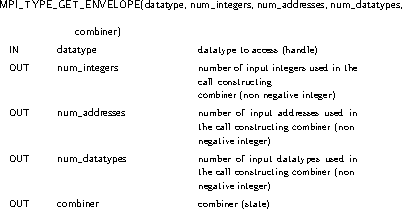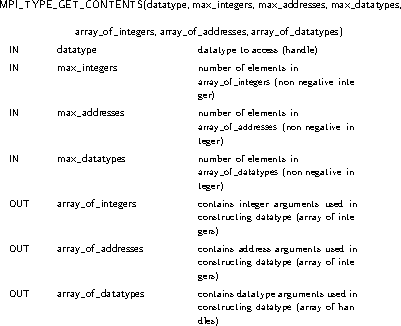## 85. Decoding a DatatypeUp: Derived Datatypes Next: Examples Previous: Correct Use of Addresses

MPI datatype objects allow users to specify an arbitrary layout of data in memory. There are several cases where accessing the layout information in opaque datatype objects would be useful. The opaque datatype object has found a number of uses outside MPI. Furthermore, a number of tools wish to display internal information about a datatype. To achieve this, datatype decoding functions are provided. The two functions in this section are used together to decode datatypes to recreate the calling sequence used in their initial definition. These can be used to allow a user to determine the type map and type signature of a datatype.int MPI_Type_get_envelope(MPI_Datatype datatype, int *num_integers, int *num_addresses, int *num_datatypes, int *combiner)

MPI_Type_get_envelope(datatype, num_integers, num_addresses, num_datatypes, combiner, ierror)
TYPE(MPI_Datatype), INTENT(IN) :: datatype
INTEGER, INTENT(OUT) :: num_integers, num_addresses, num_datatypes, combiner
INTEGER, OPTIONAL, INTENT(OUT) :: ierror
MPI_TYPE_GET_ENVELOPE(DATATYPE, NUM_INTEGERS, NUM_ADDRESSES, NUM_DATATYPES, COMBINER, IERROR)
INTEGER DATATYPE, NUM_INTEGERS, NUM_ADDRESSES, NUM_DATATYPES, COMBINER, IERROR

For the given datatype, MPI_TYPE_GET_ENVELOPE returns information on the number and type of input arguments used in the call that created the datatype. The number-of-arguments values returned can be used to provide sufficiently large arrays in the decoding routine MPI_TYPE_GET_CONTENTS. This call and the meaning of the returned values is described below. The combiner reflects the MPI datatype constructor call that was used in creating datatype.

Rationale.

By requiring that the combiner reflect the constructor used in the creation of the datatype, the decoded information can be used to effectively recreate the calling sequence used in the original creation. This is the most useful information and was felt to be reasonable even though it constrains implementations to remember the original constructor sequence even if the internal representation is different.

The decoded information keeps track of datatype duplications. This is important as one needs to distinguish between a predefined datatype and a dup of a predefined datatype. The former is a constant object that cannot be freed, while the latter is a derived datatype that can be freed. ( End of rationale.)
The list in Table 4 has the values that can be returned in combiner on the left and the call associated with them on the right.

 MPI_COMBINER_NAMED a named predefined datatype MPI_COMBINER_DUP MPI_TYPE_DUP MPI_COMBINER_CONTIGUOUS MPI_TYPE_CONTIGUOUS MPI_COMBINER_VECTOR MPI_TYPE_VECTOR MPI_COMBINER_HVECTOR MPI_TYPE_CREATE_HVECTOR MPI_COMBINER_INDEXED MPI_TYPE_INDEXED MPI_COMBINER_HINDEXED MPI_TYPE_CREATE_HINDEXED MPI_COMBINER_INDEXED_BLOCK MPI_TYPE_CREATE_INDEXED_BLOCK MPI_COMBINER_HINDEXED_BLOCK MPI_TYPE_CREATE_HINDEXED_BLOCK MPI_COMBINER_STRUCT MPI_TYPE_CREATE_STRUCT MPI_COMBINER_SUBARRAY MPI_TYPE_CREATE_SUBARRAY MPI_COMBINER_DARRAY MPI_TYPE_CREATE_DARRAY MPI_COMBINER_F90_REAL MPI_TYPE_CREATE_F90_REAL MPI_COMBINER_F90_COMPLEX MPI_TYPE_CREATE_F90_COMPLEX MPI_COMBINER_F90_INTEGER MPI_TYPE_CREATE_F90_INTEGER MPI_COMBINER_RESIZED MPI_TYPE_CREATE_RESIZED

Table 4:  combiner values returned from  MPI_TYPE_GET_ENVELOPE

If combiner is MPI_COMBINER_NAMED then datatype is a named predefined datatype.

The actual arguments used in the creation call for a datatype can be obtained using MPI_TYPE_GET_CONTENTS.int MPI_Type_get_contents(MPI_Datatype datatype, int max_integers, int max_addresses, int max_datatypes, int array_of_integers[], MPI_Aint array_of_addresses[], MPI_Datatype array_of_datatypes[])

TYPE(MPI_Datatype), INTENT(IN) :: datatype
INTEGER, INTENT(IN) :: max_integers, max_addresses, max_datatypes
INTEGER, INTENT(OUT) :: array_of_integers(max_integers)
TYPE(MPI_Datatype), INTENT(OUT) :: array_of_datatypes(max_datatypes)
INTEGER, OPTIONAL, INTENT(OUT) :: ierror
INTEGER DATATYPE, MAX_INTEGERS, MAX_ADDRESSES, MAX_DATATYPES, ARRAY_OF_INTEGERS(*), ARRAY_OF_DATATYPES(*), IERROR

datatype must be a predefined unnamed or a derived datatype; the call is erroneous if datatype is a predefined named datatype.

The values given for max_integers, max_addresses, and max_datatypes must be at least as large as the value returned in num_integers, num_addresses, and num_datatypes, respectively, in the call MPI_TYPE_GET_ENVELOPE for the same datatype argument.

Rationale.

The arguments max_integers, max_addresses, and max_datatypes allow for error checking in the call. ( End of rationale.)
The datatypes returned in array_of_datatypes are handles to datatype objects that are equivalent to the datatypes used in the original construction call. If these were derived datatypes, then the returned datatypes are new datatype objects, and the user is responsible for freeing these datatypes with MPI_TYPE_FREE. If these were predefined datatypes, then the returned datatype is equal to that (constant) predefined datatype and cannot be freed.

The committed state of returned derived datatypes is undefined, i.e., the datatypes may or may not be committed. Furthermore, the content of attributes of returned datatypes is undefined.

Note that MPI_TYPE_GET_CONTENTS can be invoked with a datatype argument that was constructed using MPI_TYPE_CREATE_F90_REAL, MPI_TYPE_CREATE_F90_INTEGER, or MPI_TYPE_CREATE_F90_COMPLEX (an unnamed predefined datatype). In such a case, an empty array_of_datatypes is returned.

Rationale.

The definition of datatype equivalence implies that equivalent predefined datatypes are equal. By requiring the same handle for named predefined datatypes, it is possible to use the == or .EQ. comparison operator to determine the datatype involved. ( End of rationale.)

The datatypes returned in array_of_datatypes must appear to the user as if each is an equivalent copy of the datatype used in the type constructor call. Whether this is done by creating a new datatype or via another mechanism such as a reference count mechanism is up to the implementation as long as the semantics are preserved. ( End of advice to implementors.)

Rationale.

The committed state and attributes of the returned datatype is deliberately left vague. The datatype used in the original construction may have been modified since its use in the constructor call. Attributes can be added, removed, or modified as well as having the datatype committed. The semantics given allow for a reference count implementation without having to track these changes. ( End of rationale.)
In the deprecated datatype constructor calls, the address arguments in Fortran are of type INTEGER. In the preferred calls, the address arguments are of type INTEGER(KIND=MPI_ADDRESS_KIND). The call MPI_TYPE_GET_CONTENTS returns all addresses in an argument of type INTEGER(KIND=MPI_ADDRESS_KIND). This is true even if the deprecated calls were used. Thus, the location of values returned can be thought of as being returned by the C bindings. It can also be determined by examining the preferred calls for datatype constructors for the deprecated calls that involve addresses.

Rationale.

By having all address arguments returned in the array_of_addresses argument, the result from a C and Fortran decoding of a datatype gives the result in the same argument. It is assumed that an integer of type INTEGER(KIND=MPI_ADDRESS_KIND) will be at least as large as the INTEGER argument used in datatype construction with the old MPI-1 calls so no loss of information will occur. ( End of rationale.)
The following defines what values are placed in each entry of the returned arrays depending on the datatype constructor used for datatype. It also specifies the size of the arrays needed which is the values returned by MPI_TYPE_GET_ENVELOPE. In Fortran, the following calls were made:

```      PARAMETER (LARGE = 1000)
INTEGER TYPE, NI, NA, ND, COMBINER, I(LARGE), D(LARGE), IERROR
!     CONSTRUCT DATATYPE TYPE (NOT SHOWN)
CALL MPI_TYPE_GET_ENVELOPE(TYPE, NI, NA, ND, COMBINER, IERROR)
IF ((NI .GT. LARGE) .OR. (NA .GT. LARGE) .OR. (ND .GT. LARGE)) THEN
WRITE (*, *) "NI, NA, OR ND = ", NI, NA, ND, &
" RETURNED BY MPI_TYPE_GET_ENVELOPE IS LARGER THAN LARGE = ", LARGE
CALL MPI_ABORT(MPI_COMM_WORLD, 99, IERROR)
ENDIF
CALL MPI_TYPE_GET_CONTENTS(TYPE, NI, NA, ND, I, A, D, IERROR)
```
or in C the analogous calls of:

```#define LARGE 1000
int ni, na, nd, combiner, i[LARGE];
MPI_Aint a[LARGE];
MPI_Datatype type, d[LARGE];
/* construct datatype type (not shown) */
MPI_Type_get_envelope(type, &ni, &na, &nd, &combiner);
if ((ni > LARGE) || (na > LARGE) || (nd > LARGE)) {
fprintf(stderr, "ni, na, or nd = %d %d %d returned by ", ni, na, nd);
fprintf(stderr, "MPI_Type_get_envelope is larger than LARGE = %d\n",
LARGE);
MPI_Abort(MPI_COMM_WORLD, 99);
};
MPI_Type_get_contents(type, ni, na, nd, i, a, d);
```
In the descriptions that follow, the lower case name of arguments is used.

If combiner is MPI_COMBINER_NAMED then it is erroneous to call MPI_TYPE_GET_CONTENTS.

If combiner is MPI_COMBINER_DUP then

 Constructor argument C Fortran location oldtype d D(1)

and ni = 0, na = 0, nd = 1.

If combiner is MPI_COMBINER_CONTIGUOUS then

 Constructor argument C Fortran location count i I(1) oldtype d D(1)

and ni = 1, na = 0, nd = 1.

If combiner is MPI_COMBINER_VECTOR then

 Constructor argument C Fortran location count i I(1) blocklength i I(2) stride i I(3) oldtype d D(1)

and ni = 3, na = 0, nd = 1.

If combiner is MPI_COMBINER_HVECTOR then

 Constructor argument C Fortran location count i I(1) blocklength i I(2) stride a A(1) oldtype d D(1)

and ni = 2, na = 1, nd = 1.

If combiner is MPI_COMBINER_INDEXED then

 Constructor argument C Fortran location count i I(1) array_of_blocklengths i to i[i] I(2) to I(I(1)+1) array_of_displacements i[i+1] to i[2*i] I(I(1)+2) to I(2*I(1)+1) oldtype d D(1)

and ni = 2*count+1, na = 0, nd = 1.

If combiner is MPI_COMBINER_HINDEXED then

 Constructor argument C Fortran location count i I(1) array_of_blocklengths i to i[i] I(2) to I(I(1)+1) array_of_displacements a to a[i-1] A(1) to A(I(1)) oldtype d D(1)

and ni = count+1, na = count, nd = 1.

If combiner is MPI_COMBINER_INDEXED_BLOCK then

 Constructor argument C Fortran location count i I(1) blocklength i I(2) array_of_displacements i to i[i+1] I(3) to I(I(1)+2) oldtype d D(1)

and ni = count+2, na = 0, nd = 1.

If combiner is MPI_COMBINER_HINDEXED_BLOCK then

 Constructor argument C Fortran location count i I(1) blocklength i I(2) array_of_displacements a to a[i-1] A(1) to A(I(1)) oldtype d D(1)

and ni = 2, na = count, nd = 1.

If combiner is MPI_COMBINER_STRUCT then

 Constructor argument C Fortran location count i I(1) array_of_blocklengths i to i[i] I(2) to I(I(1)+1) array_of_displacements a to a[i-1] A(1) to A(I(1)) array_of_types d to d[i-1] D(1) to D(I(1))

and ni = count+1, na = count, nd = count.

If combiner is MPI_COMBINER_SUBARRAY then

 Constructor argument C Fortran location ndims i I(1) array_of_sizes i to i[i] I(2) to I(I(1)+1) array_of_subsizes i[i+1] to i[2*i] I(I(1)+2) to I(2*I(1)+1) array_of_starts i[2*i+1] to i[3*i] I(2*I(1)+2) to I(3*I(1)+1) order i[3*i+1] I(3*I(1)+2] oldtype d D(1)

and ni = 3*ndims+2, na = 0, nd = 1.

If combiner is MPI_COMBINER_DARRAY then

 Constructor argument C Fortran location size i I(1) rank i I(2) ndims i I(3) array_of_gsizes i to i[i+2] I(4) to I(I(3)+3) array_of_distribs i[i+3] to i[2*i+2] I(I(3)+4) to I(2*I(3)+3) array_of_dargs i[2*i+3] to i[3*i+2] I(2*I(3)+4) to I(3*I(3)+3) array_of_psizes i[3*i+3] to i[4*i+2] I(3*I(3)+4) to I(4*I(3)+3) order i[4*i+3] I(4*I(3)+4) oldtype d D(1)

and ni = 4*ndims+4, na = 0, nd = 1.

If combiner is MPI_COMBINER_F90_REAL then

 Constructor argument C Fortran location p i I(1) r i I(2)

and ni = 2, na = 0, nd = 0.

If combiner is MPI_COMBINER_F90_COMPLEX then

 Constructor argument C Fortran location p i I(1) r i I(2)

and ni = 2, na = 0, nd = 0.

If combiner is MPI_COMBINER_F90_INTEGER then

 Constructor argument C Fortran location r i I(1)

and ni = 1, na = 0, nd = 0.

If combiner is MPI_COMBINER_RESIZED then

 Constructor argument C Fortran location lb a A(1) extent a A(2) oldtype d D(1)

and ni = 0, na = 2, nd = 1.Up: Derived Datatypes Next: Examples Previous: Correct Use of Addresses